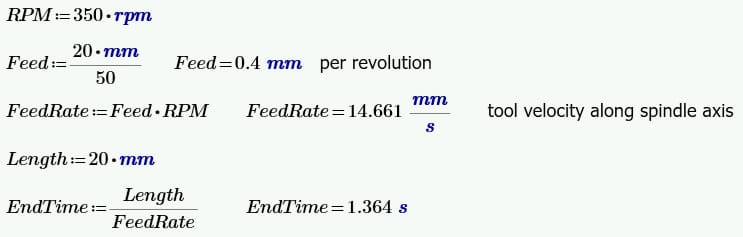Kp_9079
SubscriberRespected sir nActually i am bit confused with calculation which you shared with nwhere nfeed rate = feed *rpm, where feed = 0.4mm/revolution and rpm =350 nso according i am getting feed rate to be 2.33 mm/sec nAnd if i use the same formula and convert rpm to rad/sec that is 36.63 rad/sec then i get the same ans which you shared that is 14.661 mm/sec nso sir i have to use rpm in the formula or rad/secnpl n